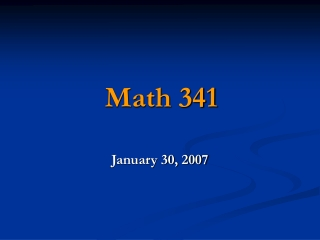Download PresentationMath 341

# Math 341 - PowerPoint PPT PresentationDownload Presentation## Math 341

- - - - - - - - - - - - - - - - - - - - - - - - - - - E N D - - - - - - - - - - - - - - - - - - - - - - - - - - -
##### Presentation Transcript

1. Math 341 January 30, 2007

2. Terminologies in Probability • Experiment – Any process that generates data. • Example: tossing a coin, rolling dice, picking a card, doing a survey, conducting experimental studies. • Sample Space – Set of all possible outcomes. • Tossing a coin: S1 = {H, T}. • Tossing a coin 3 times: S2 = {HHH, HHT, HTH, THH, HTT, THT, TTH, TTT}. • Rolling a die: S3 = {1, 2, 3, 4, 5, 6} • Picking a card from the standard deck of cards: S4 = {A♥, 2♥,…, 10♥, J♥, Q♥, K♥, A♦, …, K♦, A♠,…, K♠, A♣, …, K♣}. Total of 52 cards

3. Terminologies in Probability • Event – A subset of the sample space. • Picking a card from the standard deck of cards: S4 = {A♥, 2♥,…, 10♥, J♥, Q♥, K♥, A♦, …, K♦, A♠,…, K♠, A♣, …, K♣}. Total of 52 cards E1 = The event of selecting a heart. = {A♥, 2♥,…, 10♥, J♥, Q♥, K♥} E2 = The event of selecting a face card. = {J♥, Q♥, K♥, J♦, Q♦, K♦, J♠, Q♠, K♠, J♣, Q♣, K♣}

4. Rolling a Pair of Dice • Event – A subset of the sample space. • Rolling a pair of dice. E3 = The event of getting doubles. ={(1,1), (2,2), (3,3), (4,4), (5,5), (6,6)} E4 = The event of getting a sum of 7. ={(1,6),(6,1),(2,5),(5,2),(3,4),(4,3)} E5 = The event of getting at least one 5. ={(1,5),(2,5),(3,5),(4,5),(5,5),(6,5),(5,6), (5,4),(5,3),(5,2),(5,1)}

5. Terminologies in Probability • Experiment – Any process that generates data. • Sample Space – Set of all possible outcomes. • Event – A subset of the sample space. • Mutually Exclusive (Disjoint) – Events A and B have no common outcome. • Probability (of an event) – The chance of this event occurring. • P(E) = no. of favorable / no. of possible

6. Axioms of Probability • Axiom 1: For any event A, P(A) ≥ 0. P(S)=1. • Axiom 2: If A1, A2, …, Ak is a finite collection of mutually exclusive events, then P(A1A2  … Ak )=∑P(A) • Axiom 3: If A1, A2, …, Ak is a infinite collection of mutually exclusive events, then P(A1A2  …)=∑P(A)

7. Properties of Probability • Complement: P(AC) =1 – P(A). • Union of Events: P(AB)=P(A)+P(B) – P(AB).

8. Homework Exercises: Sec 2.1: #2, 8, 9. Sec 2.2: #12, 15, 21, 24, 26.

9. Thank you!